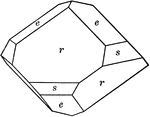Chabazite

"Hexagonal-rhombohedral. Common form is the simple rhombohedron, r, having nearly cubic angles. May…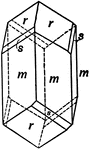Copper Silicate Showing Rhombohedral Tetartohedrism In the Hexagonal System

This is a crystal of Copper Silicate (Dioptase) with a rhombohedron of the third order, (s), in combination…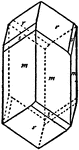Crystal of Dioptase

"...represents a crystal of dioptase with the fundamental rhombohedron (r) and the hexagonal prism of…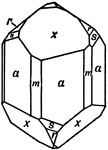Phenacite

This crystal is mainly terminated by a rhombohedron of the third order, (x). With this form are associated…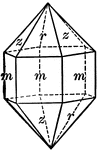Quartz

"Terminated usually by a combination of a positive and negative rhombohedron, which often are so equally…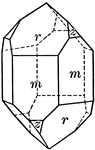Quartz

"Terminated usually by a combination of a positive and negative rhombohedron, which often are so equally…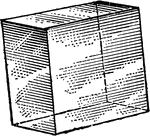Rhombohedron

"Science has succeeded in classifying the thousands of known crystals in six systems, to each of which…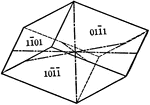Negative rhombohedron

"The rhombohedron is a form consists of six rhombic-shaped faces, which correspond in their position…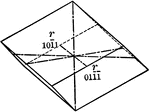Positive rhombohedron

"The rhombohedron is a form consists of six rhombic-shaped faces, which correspond in their position…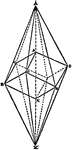Scalenohedron with inscribed Rhombohedron

"The zig-zag lateral edges [of a scalenohedron] coincide with the similar edges of a rhombohedron..."…